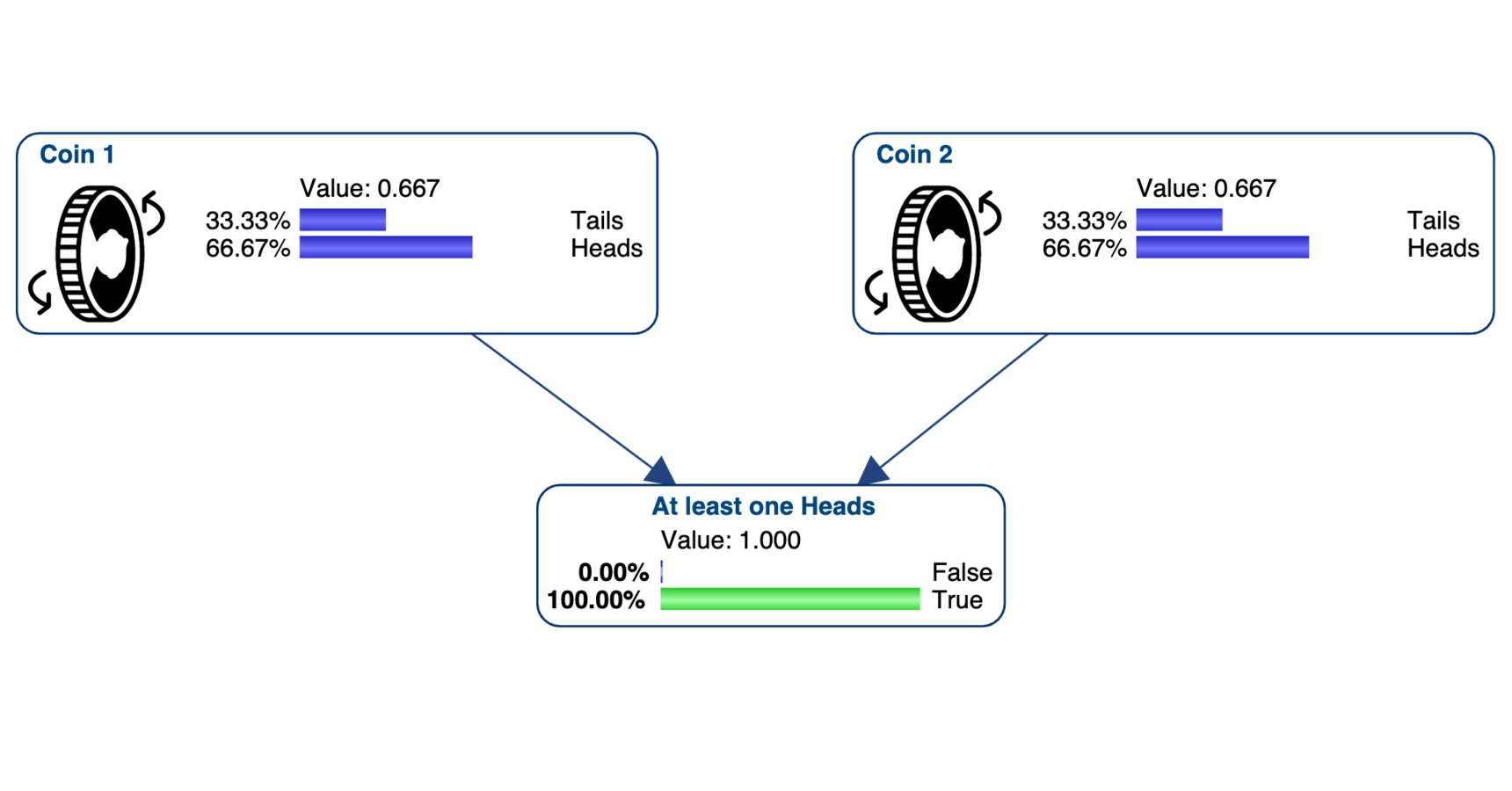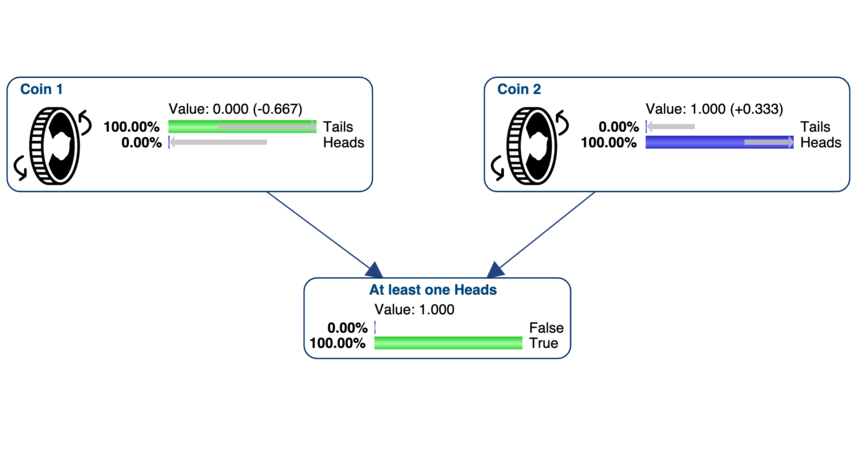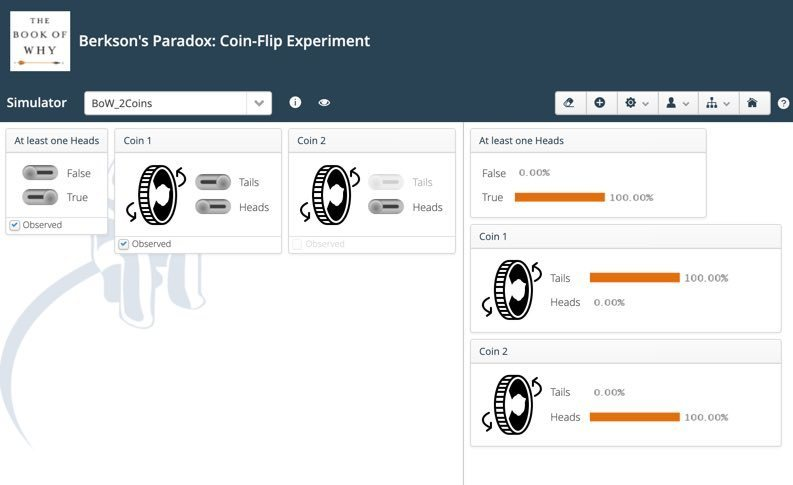0# Coin-Flip Experiment: Causal Bayesian Network"Flip two coins simultaneously one hundred times and write down the results only when at least one of them comes up heads. Looking at your table, which will probably contain roughly seventy-five entries, you will see that the outcomes of the two simultaneous coin flips are not independent. Every time Coin 1 landed tails, Coin 2 landed heads. How is this possible? Did the coins somehow communicate with each other at light speed?"

Pearl, Judea. The Book of Why: The New Science of Cause and Effect (pp. 198-199). Basic Books. Kindle Edition.

I implemented this example as a Causal Bayesian Network, which means that the arcs represent causal relationships. The two coins are the parents of the node At Least one Heads, which is the collider of this V-Structure.

The two coins are obviously marginally independent, but they become dependent when we set evidence on the collider. Writing down the results only when at least one of the coins comes up heads is equivalent to setting At Least one Heads =True.

When you then set Coin 1 = Tails, this explains away At Least one Heads =True  and set the probability of Coin 2 = Heads to 100%.You can also use the WebSimulator to experiment with this collider bias:Even though this collider bias may not seem surprising in this example (we explicitly chose to condition on the collider), on many occasions we analyze data sets where this type of selection has been made unconsciously, inducing spurious relationships between variables. This was the case in Joseph Berkson' study.